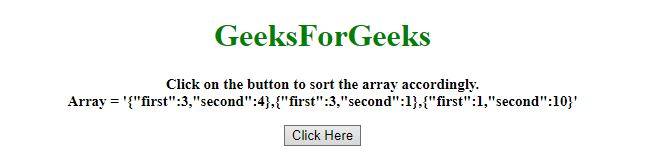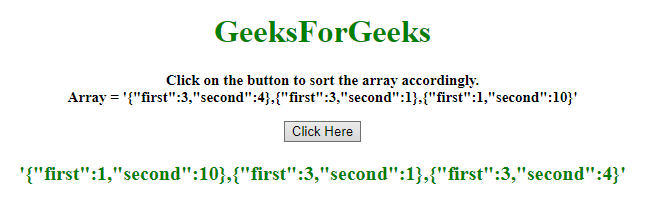# How to sort an array of object by two fields in JavaScript ?

Given an array of objects and the task is to sort the array elements by 2 fields of the object. There are two methods to solve this problem which are discussed below:

Approach 1:

• First compare the first property, if both are unequal then sort accordingly.
• If they are equal then do the same for the second property.

Example: This example implements the above approach.

 `  ` `<``html``>  ` ` `  `<``head``>  ` `    ``<``title``>  ` `        ``How to sort an array of object ` `        ``by two fields in JavaScript ? ` `    `` ` `  ` ` `  `<``body` `style` `= ``"text-align:center;"``>  ` `     `  `    ``<``h1` `style` `= ``"color:green;"` `>  ` `        ``GeeksForGeeks  ` `    `` ` `     `  `    ``<``p` `id` `= ``"GFG_UP"` `style = "font-size: ` `        ``15px; font-weight: bold;"> ` `    `` ` `     `  `    ``<``button` `onclick` `= ``"gfg_Run()"``>  ` `        ``Click Here ` `    `` ` `     `  `    ``<``p` `id` `= ``"GFG_DOWN"` `style = "color:green; ` `        ``font-size: 20px; font-weight: bold;"> ` `    `` ` `     `  `    ``<``script``> ` `        ``var el_up = document.getElementById("GFG_UP"); ` `        ``var el_down = document.getElementById("GFG_DOWN"); ` `        ``var arr = [ ` `            ``{first: 3, second: 4}, ` `            ``{first: 3, second: 1}, ` `            ``{first: 1, second: 10} ` `        ``]; ` `         `  `        ``el_up.innerHTML = "Click on the button to sort " ` `                ``+ "the array accordingly.<``br``>Array = '" ` `                ``+ JSON.stringify(arr) + ", " ` `                ``+ JSON.stringify(arr) +", " ` `                ``+ JSON.stringify(arr) + "'"; ` `         `  `        ``arr.sort(function (a, b) { ` `            ``var af = a.first; ` `            ``var bf = b.first; ` `            ``var as = a.second; ` `            ``var bs = b.second; ` `             `  `            ``if(af == bf) { ` `                ``return (as < ``bs``) ? -1 : (as > bs) ? 1 : 0; ` `            ``} else { ` `                ``return (af < ``bf``) ? -1 : 1; ` `            ``} ` `        ``}); ` `         `  `        ``function gfg_Run() { ` `            ``el_down.innerHTML` `= ``"'"` `+ JSON.stringify(arr) ` `                    ``+ ", " + JSON.stringify(arr) + ", " ` `                    ``+ JSON.stringify(arr) + "'"; ` `        ``}  ` `    ``  ` `  ` ` `  ` `

Output:

• Before clicking on the button:• After clicking on the button:Approach 2:

• First compare the first property, If both are unequal then sort accordingly.
• If they are equal then do the same for the second property, this example is following the same approach but using OR Gate to reduce the code.

Example: This example implements the above approach.

 `  ` `<``html``>  ` ` `  `<``head``>  ` `    ``<``title``>  ` `        ``How to sort an array of object ` `        ``by two fields in JavaScript ? ` `    `` ` `  ` ` `  `<``body` `style` `= ``"text-align:center;"``> ` `     `  `    ``<``h1` `style` `= ``"color:green;"` `>  ` `        ``GeeksForGeeks  ` `    `` ` `     `  `    ``<``p` `id` `= ``"GFG_UP"` `style` `= ` `        ``"font-size: 15px; font-weight: bold;"``> ` `    `` ` `     `  `    ``<``button` `onclick` `= ``"gfg_Run()"``>  ` `        ``Click Here ` `    `` ` `     `  `    ``<``p` `id` `= ``"GFG_DOWN"` `style = "color:green; ` `        ``font-size: 20px; font-weight: bold;"> ` `    `` ` `     `  `    ``<``script``> ` `        ``var el_up = document.getElementById("GFG_UP"); ` `        ``var el_down = document.getElementById("GFG_DOWN"); ` `         `  `        ``var arr = [ ` `            ``{first: 3, second: 4}, ` `            ``{first: 3, second: 1}, ` `            ``{first: 1, second: 10} ` `        ``]; ` `         `  `        ``el_up.innerHTML = "Click on the button to sort " ` `                ``+ "the array accordingly.<``br``>Array = '" ` `                ``+ JSON.stringify(arr) + ", " +  ` `                ``JSON.stringify(arr) +", " + ` `                ``JSON.stringify(arr) + "'"; ` `         `  `        ``arr.sort(function (a, b) {  ` `            ``return a.first - b.first || a.second - b.second; ` `        ``}); ` `         `  `        ``function gfg_Run() { ` `            ``el_down.innerHTML = "'" + JSON.stringify(arr) ` `                    ``+ ", " + JSON.stringify(arr) + ", " ` `                    ``+ JSON.stringify(arr) + "'"; ` `        ``}  ` `    ``  ` `  ` ` `  ` `

Output:

• Before clicking on the button:• After clicking on the button:My Personal Notes arrow_drop_upCheck out this Author's contributed articles.

If you like GeeksforGeeks and would like to contribute, you can also write an article using contribute.geeksforgeeks.org or mail your article to contribute@geeksforgeeks.org. See your article appearing on the GeeksforGeeks main page and help other Geeks.

Please Improve this article if you find anything incorrect by clicking on the "Improve Article" button below.